## Tamilnadu Samacheer Kalvi 11th Chemistry Notes Chapter 9 Solutions Notes

Solution – It is a homogeneous mixture of two or more substances consisting of atoms, ions or molecules.

Solvent – The compound that is present in large amount in a homogeneous mixture is called solvent.

Solute – The compound that is present in small amount in a homogeneous mixture is called solute.

Aqueous solution – If the water is used as the solvent the resultant solution is called an aqueous solution.

Non-aqueous solution – If solvent other than water is used, then the resultant solution is called non-aqueous solution.

Types and examples of solution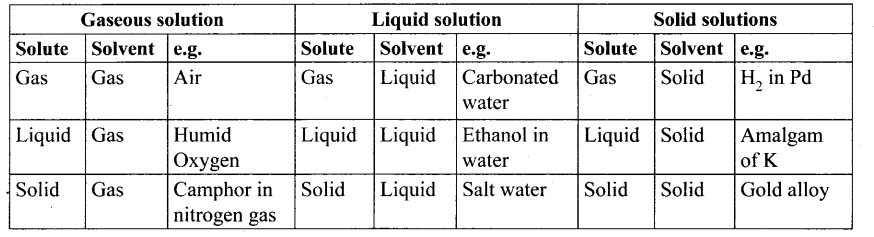Molality (m) – It is defined as the number of moles of solute present in 1 kg of the solution.

Molarity (M) – It is defined as the number of moles of solute present in 1 L of the solution.

Normality (N) – It is defined as the number of gram equivalents of solute present in 1 L of the solution.

Formality (F) – It is defined as the number of formula weight of the solute present in 1 L of the solution.

Mole fraction – It is defined as the ratio of the number of moles of the component to the total number of moles of all components present in the solution.

Mass percentage – It is the ratio of percentage of the mass of the solute to the mass of solution in grams.

Volume percentage – It is the ratio of percentage of volume of the solute to the volume of the solution in ml.

Mass by volume – It is the ratio of percentage of mass of the solute in g to the volume of the solution in ml.

Parts per million (ppm) – One ppm is equivalent to 1 milligram of the component per litre of solution.

Standard solution – A standard solution is a solution whose concentration is accurately known.

Solubility of a substance – The solubility of a substance is defined as the amount of the solute that can be dissolved in lOOg of the solvent at a given temperature to form a saturated solution.

Factors influencing the solubility – (i) Nature of solute and solvent, effect of temperature, effect of pressure.

Henry’s law – This law states that “the partial pressure of the gas in vapour phase (vapour pressure of the solute) is directly proportional to the mole fraction (x) of the gaseous solute in the solution at low concentration.
PSolute ∝ x solute in solution (or) Psolute = KH .x solute in solution.

KH – ft is the empirical constant with dimensions of pressure.

Evaporation – The process in which the liquid in converted to vapour.

Condensation – The process in which the vapour is converted to liquid.

Vapour pressure – It is defined as the pressure of vapour in equilibrium with its liquid at a given temperature.

Binary solution – The solution which contains only two components is called binary solution.

Raoult’s law – This law states that “in the case of a solution of volatile liquids, the partial pressure of each component (A & B) of the solution is directly proportional to its mole fraction.
PA = K . XA

Dalton’s law of partial pressure – This law states that the total pressure in a closed vessel will be equal to the sum of the partial pressures of the individual components.
pTotal = PA + PB

Ideal solution – It is a solution in which each component i.e., the solute as well as the solvent obeys the Raoult’s law over the entire range of concentration.

Non-ideal solutions – The solutions which do not obey Raoult’s law over the entire range of concentration are called non-ideal solution.

Factors responsible for deviation from Raoult’s law – Solute-solvent interaction, dissociation of solute, association of solute, temperature, pressure, concentration.

Colligative properties – The properties of the solution which do not depend on the chemical nature of the solute particles but depends only on the number of solute particles present in the solution are called colligative properties.

Relative lowering of vapour pressure – It is defined as the ratio of lowering of vapour pressure to vapour pressure of the solvent. Mathematically,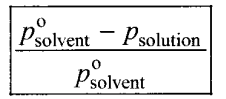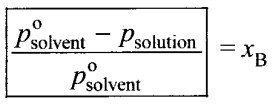Relative lowering of vapour pressure = Moles fraction of solute

Boiling point – It is the temperature at which the vapour pressure of the solution becomes equal to the atmospheric pressure (1 atm).

Elevation of boiling point – ΔTb – Tb – T0b
ΔTb = kb . m where kb = molal boiling point elevation constant.

kb Ebullioscopic constant
$$k_{b}=\frac{\mathrm{RT}_{b}^{2} \mathbf{M}_{\text {solvent }}}{\Delta \mathrm{H}_{\text {vaporisation }}}$$

Freezing point – It is defined as the temperature at which the solid and the liquid states of the substances have the same vapour pressure.

Depression in freezing point ΔTf – ΔTf = kf. m where kf = molal freezing point depression constant.

kf Cryoscopic constant.

Osmosis – It is a spontaneous process by which the solvent molecules pass through a semipermeable membrane from a solution of low concentration to a solution of higher concentration.

Osmotic pressure – The pressure that must be applied to the solution to stop the influx of the solvent (to stop osmosis) through a semipermeable membrane is called osmotic pressure.
π = CRT

Isotonic solutions – Two solutions having same osmotic pressure at a given temperature are called isotonic solutions.

Reverse osmosis (RO) – It can be defined as a process in which a solvent passes through a semipermeable membrane in the opposite direction of osmosis, when subjected to a hydrostatic pressure greater than the osmotic pressure.

van’t Hoff factor (i) – It is defined as the ratio of the actual molar mass to the abnormal molar mass of the solute.

αdissociation = $$\frac{i-1}{n-1}$$

αassociation = $$\frac{(1-i) n}{n-1}$$

Samacheer Kalvi 11th Chemistry Notes

## Tamilnadu Samacheer Kalvi 11th Chemistry Notes Chapter 10 Chemical Bonding Notes

Chemical bonds – The interatomic forces which holds the constituent atoms/ions together in a molecule are called chemical bonds.

Kossel-Lewis approach – Elements other than noble gases, try to attain the completely filled electronic configuration by losing, gaining or sharing one or more electrons from their outer shell.

Octet rule – The atoms transfer or share electrons so that all atoms involved in chemical bonding obtain 8 electrons in their outer shell.

Covalent bond – This type of mutual sharing of one or more pairs of electrons between two combining or more pairs of electrons between two combining atoms results in the formation of a chemical bond called a covalent bond.

Formal charge – Formal charge of an atom in a molecule is the electrical charge difference between the valence electron in an isolated atom and the number of electrons assigned to that atom in the lewis structure.
Formal charge –
$$\mathrm{N}_{\mathrm{V}}-\left[\mathrm{N}_{l}+\frac{\mathrm{N}_{b}}{2}\right]$$

Ionic bond (or) electrovalent bond – The bond formed by the complete transfer of electrons leads to the formation of cation and an anion which are held together by the electrostatic attractive force.

Coordinate covalent bond – In the bond formation, one of the combining atoms donates a pair of electrons and these electrons are shared by both the combining atoms. These type of bonds are called coordinate covalent bond.

Bond length – The distance between the nuclei of the two covalently bonded atoms is called bond length.

Bond order – The number of bonds formed between the two bonded atoms in a molecule is called the bond order.

Bond angle – A fixed angle created by the directional nature of the two covalent bonds which is between the two bonds in a molecule is called bond angle.

Bond enthalpy – It is defined as the minimum amount of energy required to break one mole of a particular bond in the molecule in their gaseous state.

Resonance – The lewis structures which differ only in the position of bonding and lone pair of electrons are called resonance structures and this phenomenon is called resonance.

Dipole moment – µ = q x 2d, where q = charge, 2d= distance between the two charges.

Unit of dipole moment – Debye unit (D), 1 Debye = 3.336 x 10-30 C m (C m = coulomb metre).

Non polar molecules – Molecules that have zero dipole moment are called non polar molecules. For example, H2, O2, F2.

Polar molecules – Molecules that have dipole moment value are called polar molecules. For example, HF, HCl, NO, CO.

Electronegativity difference

If XA – XB = 1.7, the bond A – B has 50% ionic character
IF XA -XB > 1.7, the bond A – B > 50% ionic character
IF XA – XB < 1.7, the bond A – B < 50% ionic character

Polarisation – A distortion in the electron cloud of the anion and its electron density drift towards the cation which results in some sharing of the valence electrons between these ions. Thus, a partial covalent character is developed between them. This phenomenon is called polarisation.

Fajan’s rule – The extent of polarisation in an ionic compound is given by Fajan’s rule.

• To show greater covalent character, both the cation and anion should have high charge on them.
• The smaller cation and larger anion show greater covalent character due to the greater extent of polarisation.
• Cation having ns2 np6 nd10 configuration show greater polarising power than the cations with ns2np(> configuration. Hence, they show great covalent character.

VSEPR theory – Valence Shell Electron Pair Repulsion Theory: It is useful in predicting the shape of the molecules.

The repulsive interaction between the electron pairs – lp – lp > lp – bp > bp – bp lp = long pair, bp = bond pair

Shapes of molecules predicted by VSEPR theory –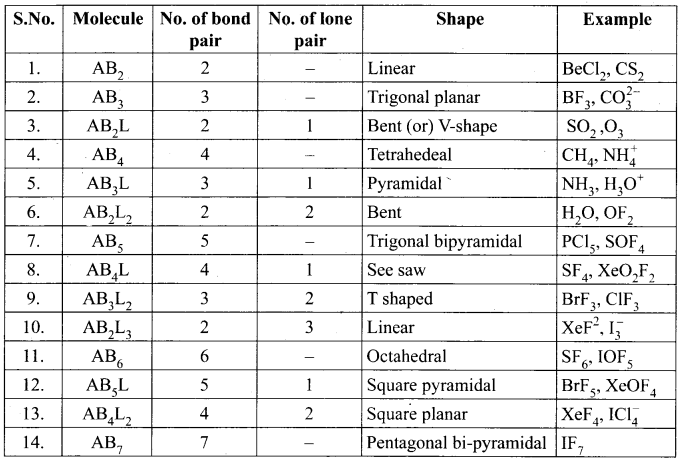Sigma bond (σ) – When two atomic orbitals overlap linearly along the axis, the resultant bond is called a bond.

Pi bond (π) – When two atomic orbitals overlap sideways, the resultant covalent bond is called pi bond (n bond).

Hybridisation – It is a process of mixing of atomic orbitals of the same atom with comparable energy to form equal number of new equivalent orbitals with same energy.

Types of hybridisation with shape and examples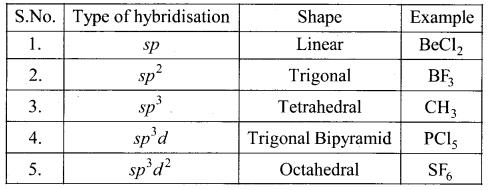Bond order – It is the half difference of number of electrons present in bonding molecular orbitals and the number of electrons present in antibonding molecular orbitals.
Bond order = $$\frac{\mathrm{N}_{b}-\mathrm{N}_{a}}{2}$$

LCAO – Linear combination of atomic orbitals.

ψ bonding – ψA + ψB

ψ antibonding – ψA ψB

Metallic bonding – The forces that keep the atoms of the metal so closely in a metallic crystal constitute is known as metallic bond (or) electronic bond.

Samacheer Kalvi 11th Chemistry Notes

## Tamilnadu Samacheer Kalvi 11th Chemistry Notes Chapter 11 Fundamentals of Organic Chemistry Notes

Organic chemistry – It is the study of compounds of carbon.

Catenation – The tendency of an atom to form a chain of bonds with the atoms of the same element is called catenation.

Functional group – It is an atom or a specific combination of bonded atoms that react in a irrespective of the organic molecule in which it is present.

Homologous series – A series of organic compounds each containing a characteristic functional group and the successive members differ from each other in a molecular formula by a CH2 group is called homologous series.

Alkanes – CnH2n + 2

Alkenes – CnH2n

Alkynes- CnH2n-2

IUPAC – International Union of Pure and Applied Chemistry.

Nuclear substituted aromatic compound – These are the compounds in which the functional group is directly attached to the benzene ring. These are derivatives of benzene.

Molecular formula of an organic compound – It is the simplest, least informative representation, showing the ratio of atoms present.

Structural formula of an organic compound – It is the formula that shows the way of atoms in a compound comiected to each other.

Molecular models – These are physical devices that are used for a better visualisation and perception of three dimensional shapes of organic molecules.

Three dimensional representation of organic molecule – The simplest convention is solid and dashed wedge formula in which 3-D image of a molecule can be perceived from two dimensional picture.

Fisher projection formula – This s a method of representing three dimensional structures in two dimension.

Saw horse projection formula – The bond between two carbon atoms is drawn diagonally and slightly elongated. The lower left hand carbon is considered lying towards the front and the upper right hand carbon is considered lying towards the back.

Newman projection formula – In this formula, the molecules are viewed from the front along the carbon-carbon bond axis.

Isomerism – It is a phenomenon in which two or more compounds with the same molecular formula but different structures and properties are there.

Isomers – Compounds exhibiting the isomerism are called isomers.

Constitutional isomers – These isomers have same molecular formula but differ in their bonding sequence.

Chain (or) nuclear (or) skeletal isomerism – These isomers differ in the way in which the carbon atoms are bonded to each other in a carbon chain.

Position isomerism – Compounds with the same molecular formula and carbon skeleton but differ in the position of substituent are said to exhibit position isomerism.

Functional isomerism – Different compounds having the same molecular formula but different functional groups are said to exhibit functional isomerism.

Metamerism – It is a special kind of structural isomerism arises due to the unequal distribution of carbon atoms on either side of the functional group or different alkyl groups attached to the either side of the same functional group and having the same molecular formula.

Tautomerism – It is a special type of functional isomerism in which a single compound exists in two readily interconvertible structures that differ markedly in the relative position of atleast one atomic nucleus, generally hydrogen.

Stereoisomerism – The phenomenon in which the isomers which have same bond connectivity but different arrangement of groups or atoms in space in known as stereoisomerism.

Geometrical isomerism – The phenomenon in which the stereoisomers which have different arrangement of groups or atoms around a rigid framework of double bonds.

Cis isomer – It is the one in which two similar groups are on the same side of the double bond.

Trans isomer – It is the one in which two similar groups are on the opposite side of the double bond.

Optical isomerism – Compounds having same physical and chemical property but differ only in the rotation of plane of the polarised light are known as optical isomers and the phenomenon is known as optical isomerism.

Dextro rotatory – The optical isomer which rotates the plane polarised light to the right or in clockwise direction is said to be dextro rotatory.

Leavo rotatory – The optical isomer which rotates the plane polarised light to the left or in . anti-clockwise direction is said to be leavo rotatory.

Enantiomers – The optical isomers which rotate the plane polarised light with equal angle but in opposite direction are known as enantiomers.

Chiral carbon – A carbon atom whose tetravalency is satisfied by four different substituents is called asymmetric carbon or chiral carbon.

Sublimation – The process in which the substances on heaving converted directly from solid to vapour without melting is known as sublimation.

Azeotropes – Compounds which are constant boiling mixtures.

Extraction – The process of removing a substance from its aqueous solution by shaking with a suitable organic solvent is termed extraction.

Chromatography – It is selective distribution of the mixture of organic substances between a stationary phase and a moving phase (or) chromatography is defined as a technique for the separation of a mixture brought about by differential movement of the individual compound through porous medium under the influence of moving solvent.

$$R_{f}=\frac{\text { Distance moved by the substance from base line }(X)}{\text { Distance moved by the solvent from base line }(Y)}$$

Samacheer Kalvi 11th Chemistry Notes

## Tamilnadu Samacheer Kalvi 11th Chemistry Notes Chapter 12 Basic Concepts of Organic Reactions Notes

Organic reactions – Substrate is an organic molecule reacts with reagent, which may be an organic, inorganic or any agent like heat, photon etc that brings about the chemical change to form a product. This is known as organic reactions.

Mechanism of the reaction – The series of simple steps which collectively represent the chemical change, from substrate to product is called as the mechanism of the reaction.

Type of fission of a covalent bonds – (i) Homolytic fission, and (ii) Heterolytic fission.

Homolytic cleavage –

• Homolytic cleavage is the process in which a covalent bond breaks symmetrically in such way that each of the bonded atoms retains one electron.
• This type of cleavage occurs under high temperature or in the presence of UV-light.
• In such molecules, the cleavage of bonds results into free radicals.
• Free radicals are short lived and highly reactive species.

Free radical initiators – The type of reagents that promote homolytic cleavage in substrate are called as free radical initiators.

• Azobisisobutyronitrile (AIBN)
• Benzoyl peroxide

Heterolytic cleavage –

• Heterolytic cleavage is the process in which a covalent bond breaks unsymmetrically such that one of the bonded atoms retains the bonded pair of electrons.
• It results in the formation of a cation and an anion.

Carbocation – In a carbocation, the carbon atom bearing positive charge. It is sp2 hybridised and hence it has a planar structure.

Carbanion – In a carbanion,, the carbon atom bearing negative charge. It is sp3 hybridised and hence it is pyramidal in shape.

Nucleophiles – Nucleophiles are reagents that has high affinity for electropositive centers. They possess an atom has an unshared pair of electrons. They are usually negatively charged ions or electron rich neutral molecules.

Nucleophilic reagents – Ammonia, amines, water, alcohols, ethers, hydrogen sulphide, thiols.

Electrophiles – Electrophiles are reagents that are attracted towards negative charge or electron rich center. They are either positively charged ions or electron deficient neutral molecules.

Electrophilic reagents – Carbon dioxide, Aluminium chloride, borontrifluoriuc and ferric chloride.

Electron movement in organic reactions – There are three types of electron movement,

1. Lone pair becomes a bonding pair.
2. Bonding pair becomes a lone pair.
3. a bond breaks and becomes another bond.

Electron displacement effects in covalent bonds – Electron displacement effects in covalent bonds occurs due to the presence of an atom or group of different electronegativity or under the influence of some outside attaching group. The electron displacements are categorized into, Inductive effects, Resonance effect, electromeric effect and hyper conjugation.

Inductive effect (I) – It is defined as the change in the polarization of a covalent bond due to the presence of adjacent bonded atoms or groups in the molecule.

+1 effect – Atoms or groups which lose electron towards a carbon atoms are said to have a +1 effect. Example, (CH3)3C-, (CH3)2CH-, CH3-CH2-, CH3

-I effect – Atoms or groups which draw electrons away from a carbon atom one said to have a -I effect. Example, -NO2, F, Cl, Br, I , -OH, C6H5

Electromeric effect – The electromeric effect refers to the polarity produced in a multiple bonded compound when it is attacked by a reagent when a double or a triple bond is exposed to an attack by an electrophile the two n electrons which from the 7t bond are completely transferred to one atom or the other.

Resonance or mesomeric effect – Certain organic compounds can be represented by more than one structure and they differ only in the position of bonding and lone pair of electrons. Such structure are called resonance (or) canonical structure. This phenomenon is also called resonance effect.

Positive resonance effect – Those atoms or groups which lose electrons towards a carbon atom are said to have a + M or + R effect.

Example, -Cl, -Br, -I, -NH2, -NR2, -OH, -OCH2

Negative resonance effect – Those atoms or groups which draw electrons away from a carbon atom are said to have a -M or -R effect.

Example: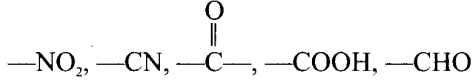Hyper conjugation – The delocalization of electron of a-bond is called as hyper conjugation.

Substitution reaction – In this reaction an atom or a group of atoms attached to a carbon atom is replaced by a new atom or a groups of atoms.

Addition reaction – It is a characteristic reaction of an unsaturated compound. In this reaction two molecules combine to give a single product.

Elimination reaction – In this reaction two substituents are eliminated from the molecule, and a new C-C double bond is formed between the carbon atoms to which the eliminated atoms\groups are previously attached.

Oxidation and reduction reactions – Most of the oxidation reaction of organic compounds involves gain of oxygen or loss of hydrogen. Reduction involves gain of hydrogen and loss of oxygen.

Functional group inter conversion – Organic synthesis involves functional group inter conversions. A particular functional group can be converted into other functional group by reacting it with suitable reagents.

Samacheer Kalvi 11th Chemistry Notes

## Tamilnadu Samacheer Kalvi 11th Chemistry Notes Chapter 13 Hydrocarbons Notes

Alkenes – Alkenes are unsaturated hydrocarbons that contain carbon-carbon double bond. They are represented by the general formulae CnH2n where n stands for the number of carbon atoms in the molecule. Alkenes are also known as olefins.

Geometrical isomerism – It is a type of stereoisomerism and it is also called cis-trans isomerism or geometrical isomerism. Such type of isomerism results due to the restricted rotation of doubly bonded carbon atoms.

MarkovnikofFs rule – When an unsymmetrical alkene reacts with hydrogen halide, the hydrogen atom adds to the carbon that has more number of hydrogen atoms and halogen adds to the carbon atom having fewer hydrogen atoms.

Polymerisation – A polymer is a large molecule formed by the combination of large number of small molecules. The process is known as polymerisation.

Alkynes – Alkynes are unsaturated hydrocarbons that contain carbon-carbon triple bond in their molecules. Their general molecular formula is CnH2n-2.

Ozonolysis – Ozone adds to carbon-carbon triple bond of alkynes to form ozonides. This process is known as ozonolysis.

Aromaticity – A compound may be aromatic, if it obey the following rules,
The molecule must be co-planar.
Complete delocalisation of π electrons in the ring.
Presence of (An + 2)π electrons in the ring, where n is an integer (n = 0,1,2 …). This is known as Huckel’s rule.

Ortho and para directing groups – OH, – NH2, – NHR, – CH3 – OCH3 etc.

Meta directing groups NO2, – CN, – CHO, – COOH etc.

Polynuclear aromatic hydrocarbons – Two or more benzene rings fused to form polynuclear aromatic hydrocarbons.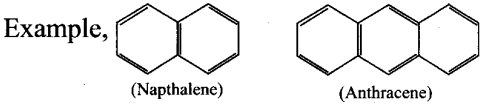Samacheer Kalvi 11th Chemistry Notes

## Tamilnadu Samacheer Kalvi 11th Chemistry Notes Chapter 14 Haloalkanes and Haloarenes Notes

Haloalkanes – When one or more hydrogen atoms of aliphatic hydrocarbons are replaced by a halogen atom, the resultant compounds are called Haloalkanes.

Haloarene – When one or more hydrogen atoms of aromatic hydrocarbons are replaced by a halogen atom , the resultant compounds are called Haloarenes.

R-X – Mono halogen derivatives of alkanes are called haloalkanes. They are classified as 1° (Primary), 2° (secondary) and 3° (Tertiary) haloalkanes.

Nature of C-X bond in haloalkanes – Carbon halogen bond is a polar bond as halogens are more electronegative than carbon.

Ammonolysis – Reaction with alcoholic ammonia:

Ambident Nucleophile – Nucleophiles such as cyanide and nitrite ion which can attack the nucleophilic centre from two sides are called ambident nucleophiles..

SN2 Bimolecular nucleophilic substitution reactions.

SN1 Unimolecular nucleophilic substitution reactions.

E2 reaction – Bimolecular elimination reactions.

E1 reaction – Unimolecular elimination reactions.

Organometallic compounds – They are organic compounds in which there is a direct carbon-metal bond, e.g., CH3MgI Methyl magnesium iodide.

Haloarene – C6H5-X: In this type of compounds atoms is directly attached to benzene ring.

Resonance Structure of Halobenzene –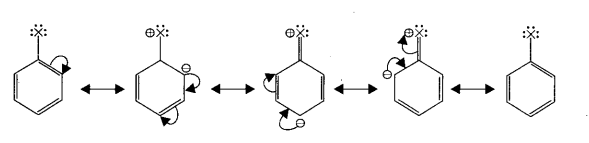Polyhalogen compounds – Carbon compounds containing more than one halogen atoms are called polyhalogen compounds.

Gemdihalide – If two halogen atoms are attached to one carbon atom, it is called gem dihalide, eg., CH3CHCl2-Ethylidene chloride.

Vicinal dihalide – If two halogen atoms are attached to first two carbon atoms, it is called vicinal dihalide.

CHCl3 It is chloroform.

CCl4 It is tetrachloromethane (or) Carbontetrachloride.

Freops – (CFC’s) The chlorofluoro derivatives of methane and ethane are called freons.

DDT – It stands for p,p’ – dichloro diphenyl trichloroethane. It is an organic pesticide.

Samacheer Kalvi 11th Chemistry Notes

## Tamilnadu Samacheer Kalvi 11th Chemistry Notes Chapter 15 Environmental Chemistry Notes

Environment – It includes the air we breathe, the water that covers most of the earth’s surface, the plants and animals around us and much more.

Environmental chemistry – It is the study of chemicals and chemical processes.

Environmental pollution – Any desirable change in our environment that has harmful effects on plants, animals and human beings is called environmental pollution.

Pollutants – The substances which cause pollution of the environment are called pollutants.

Bio-degradable pollutants – The pollutants which can be easily decomposed by the natural biological processes are called biodegradable pollutants.

Non-biodegradable pollutants – The pollutants which cannot be decomposed by the natural biological processes are called non-biodegradable pollutants.

Air – It is a mixture of 78% nitrogen, 21% oxygen, 0.93% argon, 0.04% carbon dioxide, trace amount of other gases and a little amount of water vapour.

Troposphere – The lowest layer of the atmosphere is called the troposphere and it extends from 0-10 km from the earth’s surface.

Hydrosphere – It includes all types of water sources like oceans, seas, rivers, lakes, streams, underground water, polar ice-caps, clouds and it covers about 75% of the earth’s surface.

Lithosphere – It includes soil, rocks and mountains which are the solid components of earth.

Biosphere – It includes hydrosphere, atmosphere and lithosphere.

Air pollution – Any undesirable change in air which adversely affects living organisms is called air pollution.

Gaseous air pollutants – Oxides of sulphur, oxides of nitrogen, oxides of carbon and hydrocarbons are the.gaseous air pollutants.

Greenhouse effect – It is defined as the heating up of the earth surface due to trapping of infrared radiations reflected by earth’s surface by C02 layer in the atmosphere.

Global warming – The heating up of the earth through greenhouse effect is called global warming.

Acid rain – Oxides of sulphur and nitrogen in the atmosphere are absorbed by droplets of water that make up clouds and chemically converted into sulphuric acid nitric acid and it results in the pH of rain water to become 5.6. It is called acid rain.

Stone leprosy – The extensive damage on marble by acid rain is called stone leprosy.

Particulate pollutants – They are small size biotic and aboitic solid particles and liquid droplets suspended in air. e.g., dust, pollen, smoke. Many of them are hazardous.

Viable Particulates – They are small size living organisms such as bacteria, fungi, moulds, algae which are dispersed in air.

Non-viable particulates – They are small size solid particles and liquid droplets suspended in air e.g., smoke, dust, mist, fumes.

Pneumoconiosis – The condition in which there is irritation of lungs which causes cancer and asthma by particulate pollutants is called pneumoconiosis.

Smog – It is a combination of smoke and fog which form droplets that remains suspended in air.

Classical smog on London smog – It consists of coal-smoke and fog.

Photochemical smog or Los Angeles smog – This is formed by the combination of smoke, dust and fog along with air pollutants like oxides of nitrogen and hydrocarbons in the presences of sunlight.

PAN – It stands for peroxy acetyl nitrate

Depletion of ozone – Loss of ozone molecules in the upper atmosphere is termed as depletion of stratospheric ozone.

Freons – The chlorofluro derivates of methane and ethane are called freons.

Water pollution – It is defined as the addition of foreign substances or factors like heat which degrades the quality of water, so that it becomes health hazardous or unfit for use.

Water pollutants – Microorganisms like bacteria, Viruses and protozoa acts as water pollutants.

Algal bloom – The enhanced plant growth in water bodies is called algal bloom.

Eutrophication – The process in which the nutrient rich water bodies supports a dense plant population kills animal life by depriving it of oxygen and results in loss of biodiversity is known as eutrophication.

Biochemical oxygen demand (BOD) – The total amount of oxygen in milligrams consumed by microorganisms in decomposing the waste in one litre of water at 20°C for a period of 5 days is called biochemical oxygen demand (BOD) and its value is expressed in ppm.

Chemical oxygen demand (COD) – It is defined as the amount of oxygen required by the organic matter in a sample of water for its oxidation by a strong oxidising agent like K2Cr207 in acidic medium for a period of 2 hours.

Total dissolved solids (TDS) – It includes cations like cadmium, magnesium, sodium, potassium, iron and anions like carbonate, bicarbonate, chloride, sulphate, phosphate and nitrate.

Soil – It is a thin layer of organic and inorganic material that covers the earth’s rocky surface.

Soil pollution – It is defined as the build up of persistent toxic compounds, radioactive materials, chemical salts and disease causing agents in soils which have harmful effects on plant and animal growth.

Essential Nutrients for soil – Nitrogen, Phosphorous, Potassium. (N, P, K).

Pesticides – These are the chemicals that are used to kill or stop the growth of unwanted organisms.

Irtsectides – These are the chemicals that are used to kill the insects, e.g., DDT, BLTC, Aldrin.

Fungicides – These are used to destroy fungus, e.g., Organic mercury compounds.

Herbicides – These are the chemicals used to control the growth of unwanted plants. They are also called “weedkillers” e.g., Sodium chlorate, sodium arsenite.

Industrial wastes – It may include cyanides, chromates, acids, alkalis, and metals like Hg, Cu, Zn, Cd and Pb.

Green chemistry – It is a chemical philosophy encouraging the design of products and processes that reduce or eliminates the use and generation of hazardous substances.

World environmental day – June 5

Ozone layer protection day – 16th September

Samacheer Kalvi 11th Chemistry Notes

## Tamilnadu Samacheer Kalvi 11th Chemistry Notes Chapter 8 Physical and Chemical Equilibrium Notes

Physical equilibrium: A system in which the amount of matter constituting different phases does not change with time is said to be in physical equilibrium.

Solid-liquid equilibrium: H2O(s) ⇌ H2O(l)
At equilibrium, Rate of melting of ice = Rate of freezing of water

Liquid-vapour equilibrium: H2O(l) ⇌ H2O(g)
At equilibrium, Rate of evaporation = Rate of condensation

Solid-Vapour equilibrium: I2(s) ⇌ I2(g)

At equilibrium, Rate of sublimation = Rate of deposition

Equilibrium involving dissolution of solid in liquid: Sugar (solid) ⇌ Sugar (solution) At equilibrium, Rate of dissolution of solute = Rate of crystallisation of solute

Gas in liquid equilibrium: C2 (g) ⇌ CO2 (solution)

At equilibrium, Rate of vaporisation = Rate of condensation

Chemical equilibrium: In a chemical reaction, chemical equilibrium is the state in which both reactants and products are present in concentration which have no further tendency to change with time.

At chemical equilibrium,
Rate of forward reaction (Rf) = Rate of reverse reaction (Rr)

Dynamic equilibrium: At equilibrium, the forward and the backward reactions are proceeding at the same rate and no macroscopic change is observed. So chemical equilibrium is in a state of dynamic equilibrium.

Homogeneous equilibrium: In this equilibrium, all the reactants and products are in the same phase.
H2(g) + I2(g) ⇌ 2HI(g)

Heterogeneous equilibrium: If the reactants and products of a reaction in equilibrium are in different phases, then it is called as heterogeneous equilibrium.
CaCO3(s) ⇌ CaO (s) + CO2(g)

Law of mass action: This law states that “At any instant, the rate of a chemical reaction at a given temperature is directly proportional to the product of the active masses of the reactants at that instant.

Active mass: [n/V]mol dm-3 (or) mol L-1 where “w” is the number of moles of substance and V is the volume of the substance in dm3 or L.

Equilibrium constants Kc and Kp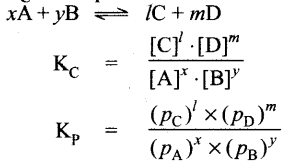Relation between Kp and KC : KP = KC (RT)Δng

Equilibrium constant for heterogeneous equilibrium:
CaCO3(s) ⇌ CaO(s) + CO2(g)
KC – [CO2], Kp – pCo2

Application of equilibrium constant: It is used to predict the direction in which the net reaction will take place, predict the extent of the reaction, calculate the equilibrium concentrations of reactants and products.

Reaction quotient, Q: Under non equilibrium conditions it is defined as the ratio of the product of active masses of reaction products raised to the respective stoichiometric coefficients in the balanced chemical equation to that of the reactants.
$$\frac{[\mathrm{C}]^{l}[\mathrm{D}]^{m}}{[\mathrm{~A}]^{x}[\mathrm{~B}]^{y}}$$
Under non equilibrium conditions.

If Q = KC, the reaction is in equilibrium state. If Q > KC, the reaction will proceed in the reverse direction, i.e., formation of reactants. If Q < KC, the reaction will proceed in the forward direction, i.e., formation of products.

Kp and Kc values for synthesis of HI: H2(g) + I2(g) ⇌ 2HI(g)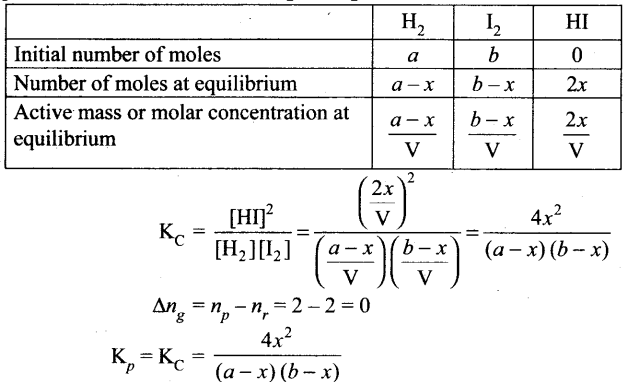Dissolution of PCl: PCl(g) ⇌ PCl3(g) + Cl2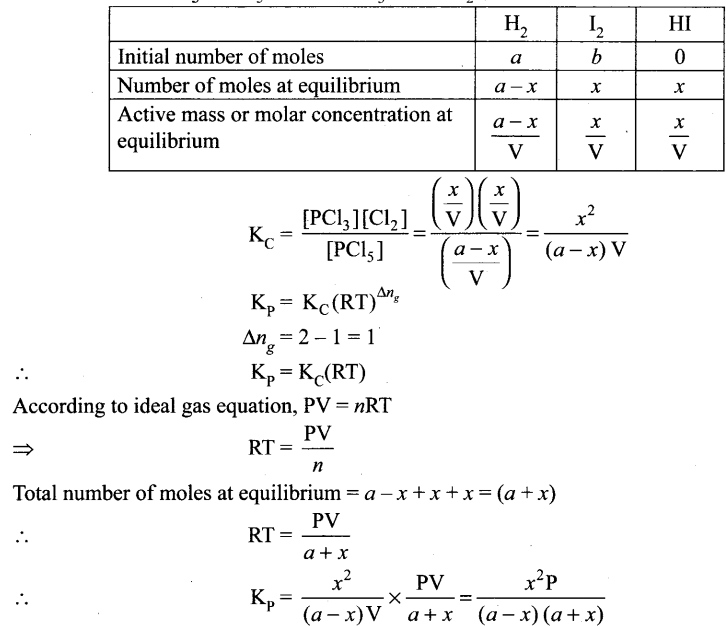Synthesis of amonia: N2(g) + 3H3(g) ⇌ 2NH3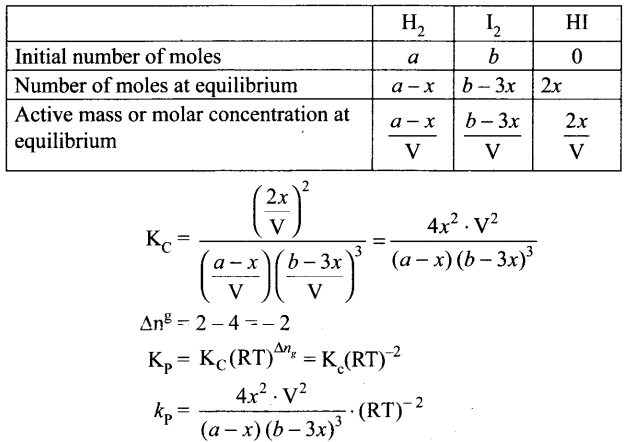Le-Chatlier’s principle: “If a system at equilibrium is disturbed, then the system shifts itself in a direction that nullifies that effect of that disturbance.”

Effect of concentration : Increase in concentration of a substance is to shift the equilibrium in a direction that consumes the added substance and vice-versa.

Effect of pressure: When pressure of an equilibrium system having gaseous components is increased then the equilibrium shift in the direction that has fewer moles of gaseous components and vice-versa.

Effect of temperature: If the temperature of an equilibrium system increases then the equilibrium shift in the direction that consumes heat and vice-versa.

Effect of catalyst: It does not affect the state of equilibrium. However, it speed up the attainment of equilibrium by lowering activation energy.

Effect of inert gas: When an inert gas is added to an equilibrium system at constant volume then it has no effect on equilibrium.

Van’t Hoff equation: ∆G° = – RT/wK and ∆G° = ∆H° – T∆S° ⇒ – RTlnK = ∆H° – T∆S° where K = equilibrium constant , ∆G° = free energy change, ∆H° = Enthalpy change, AS° = Entropy change

Differential form of van’t Hoff equation: $$\frac{d(\ln \mathrm{K})}{d \mathrm{~T}}=\frac{\Delta \mathrm{H}^{\circ}}{\mathrm{RT}^{2}}$$

Integrated form of van’t Hoff equation: $$\log \frac{\mathrm{K}_{2}}{\mathrm{~K}_{1}}=\frac{\Delta \mathrm{H}^{\circ}}{2.303 \mathrm{R}}\left[\frac{\mathrm{T}_{2}-\mathrm{T}_{1}}{\mathrm{~T}_{2} \mathrm{~T}_{1}}\right]$$

Samacheer Kalvi 11th Chemistry Notes

## Tamilnadu Samacheer Kalvi 11th Chemistry Notes Chapter 7 Thermodynamics Notes

Thermodynamics – It means flow of heat. It is a branch of science which deals the relation between energy, heat, work and accompanying changes in the nature and behaviour of various substances around us.

Macroscopic properties – Heat and work.

First law of Thermodynamics – It states that “The total energy of an isolated system remains constant though it may change from one form to another”.

ΔU = q + w
ΔU – Change in the internal energy
q – Amount of heat supplied to the system
w – Amount of work done on the system

System – It is defined as any portion of matter under thermodynamic consideration, which is separated from the rest of the universe by real or imaginary boundaries.

Homogeneous system – If the physical states of all its matter are uniform, it is called homogeneous system.

Heterogeneous system – If the physical states of all its matter are not uniform, it is called heterogeneous system.

Surroundings – Everything in the universe that is not the part of system is called surroundings.

Boundary – Anything which separates the system from its surrounding is called boundary.

Isolated system – A system which can exchange neither matter nor energy with its surroundings is called an isolated system, e.g., Hot water contained in a thermos flask.

Closed system – A system which can exchange only energy but not matter with its surroundings is called a closed system, e.g., Hot water contained in a closed beaker.

Open system – A system which can exchange both matter and energy with its surroundings is called an open system, e.g., Hot water contained in an open beaker.

Intensive properties – The properties that are independent of the mass or size of the system is called as intensive property, e.g., Refractive index, surface tension, density, temperature, boiling point and freezing point etc.

Extensive properties – The properties that are depended on the mass or size Of the system is called as extensive property, e.g., Volume, number of moles, mass and internal energy etc.

Thermodynamic process – The method of operation which can bring about the change in the system is called thermodynamic process, e.g., Heating, cooling and fusion.

Reversible process – The process in which the system and surroundings can be restored to the initial state from the final state without producing any changes in the thermodynamic properties of the universe is called a reversible process.

Irreversible process – The process in which the system and surroundings cannot be restored to the initial state from the final state is called an irreversible process.

Adiabatic process – It is defined as one in which there is no exchange of heat (q) between the system and surrounding during process, [q = 0]
304 Chemistry-11 :

Isothermal process – It is defined as one in which the temperature of the system remains constant, during the change from its initial to final state. [dT = 0]

Isobaric process – It is defined as one in which the pressure of the system remains constant during its change from the initial to final state. [dP = 0]

Isochoric process – It is defined as one in which the volume of system remains constant during its change from initial to final state. [dV= 0]

Cyclic process – When a system returns to its original state after completing a series of changes the process is known as a cyclic process. For a cyclic process dU = 0, dH = 0, dP = 0, dV= 0 and dT= 0.

State functions – The properties of P, V, T and ‘n’ that are used to describe the state of a system are called as state functions.

Path functions – A path function is a thermodynamic property of the system whose value depends on the path by which the system goes from its initial to final states, e.g., Work and heat.

Internal energy – Internal energy of a system is equal to the energy possessed by all its constituents namely atoms, ions and molecules.
U = Internal energy = Ut + Uv + Ur + Ub + Ue + Ui.

ΔU – Change in internal energy = Uf– Ui.

Heat (q) – It is regarded as an energy in transit across the boundary separating a system from its surrounding. It is a path function.

Units of heat – SI unit of heat is joule (J).The other unit is calories (cal).

Calories (cal) – It is defined as the quantity of heat required to raise the temperature of 1 gram of water by 1 °C in the vicinity of 15°C.

Work (w) – It is defined as the force (F) multiplied by the displacement (x). [- w = F. x ]

Unit of work – SI unit of work is joule (J) or kilojoule (kJ).

Joule – It is defined as the work done by a force of one Newton through a displacement of one meter (J = Nm).

Gravitational work – When an object is raised to a certain height against the gravitational field, gravitational work is done on the object, [w = m.g.h]

Electrical work – When a charged body moves from one potential region to another, electrical work is done, [w = QV]

Mechanical work – It is defined as force multiplied by the displacement through which the force acts, [w = F. x]

Pressure – Volume work – The work done in expansion or compression of a gas. PV work (or) expansion work.

Work involved in expansion and compression process – [w = -PΔV]

Expansion – During expansion, work is done by the system. Since Vf > Vi., the sign obtained for work will be negative.

Compression – During compression, work is done on the system. Since Vf < Vi the sign obtained for work will be positive.

For Reveresible process wrev = 2.303 nRT log $$\left(\frac{\mathrm{V}_{f}}{\mathrm{~V}_{i}}\right)$$

Zeroth law of thermodynamics – This law states that ‘If two systems are separately in thermal equilibrium with a third one, then they tend to be in thermal equilibrium with themselves’.

First law of thermodynamics – “Energy can neither be created nor destroyed, but may be converted from one form to another”.
(or)
The total energy of a system and surroundings remains constant (or conserved).
ΔU = q + w

Mathematical statement of first law –

 ΔU = q + w Cyclic process ΔU = 0 ∴ q = -w Isochoric process ΔV = 0 w = 0 ∴ ΔU = qv Adiabatic process Q = 0 ∴ ΔU = w Isobaric process ΔU = q + w ΔU = q – p ΔV

Enthalpy (H) – It is defined as the sum of the internal energy (U) of a system and the product of pressure and volume of the system.
H = U + PV
ΔH = ΔU + PΔV

Relation between enthalpy ‘H’ and internal energy ‘U’ –
ΔH — ΔU + Δn(g)RT

Standard heat of formation – It is defined as “the change in enthalpy that takes place when one mole of a compound is formed from its elements, present in their standard states (298K and 1 bar pressure).

Thermochemical equations – It is a balanced stoichiometric chemical equation that includes the enthalpy change (ΔH).

Standard enthalpy of reaction (ΔH0r) – It is the enthalpy change for a reaction when all the reactants and products are present in their standard states.
$$\Delta \mathrm{H}_{r}^{0}=\Sigma \Delta \mathrm{H}_{r}^{0} \text { (products) }-\Sigma \Delta \mathrm{H}_{r}^{\theta} \text { (reactants) }$$

Heat of combustion (ΔHc) – It is defined as the change in enthalpy of a system when one mole of the substance is completely burnt in excess of air or oxygen.

Specific heat capacity (C) – It is defined as the heat absorbed by one kilogram of a substance in raising its temperature by one Kelvin at a specified temperature.
$$\mathrm{C}=\left[\frac{q}{m\left(\mathrm{~T}_{2}-\mathrm{T}_{1}\right)}\right]$$

Molar heat capacity – It is defined as the amount of heat absorbed by one mole of the substance to raise its temperature by 1 Kelvin.
cm = q/ΔT

Unit of heat capacity – JK-1 mol-1

Cv : (Molar heat capacity at constant volume) – It is defined as the rate of change of internal energy with respect to temperature at constant volume.
$$C_{V}=\left(\frac{d U}{d T}\right)_{V}$$

Cp: (Molar heat capacity at constant pressure) – It is defined as the rate of change of enthalpy with respect to temperature at constant pressure.
$$C_{P}=\left(\frac{d H}{d T}\right)_{P}$$

Relation between Cp and Cv for an ideal gas –
Cp – Cv = nR

Calorimetry – It is the science associated with determining the changes in energy of a system by measuring the heat exchanged with the surroundings. ,

Exothermic reaction – The reaction in which heat is evolved and system loses heat to the surroundings. [ΔHr = -ve]

Endothermic reaction – The reaction in which heat is absorbed and system gains heat from the surroundings. [ΔHr= +ve] .

Calorific value – It is defined as the amount of heaf produced in calories (or joules) when one gram of a substance is completely burnt. The SI unit is J kg-1.

Heat of solution – It is defined as the change in enthalpy of the system when one mole of a substance is dissolved in a specified quantity of a solvent at a given temperature.

Heat of neutralisation – It is defined as the change in enthalpy of the system when one gram equivalent of an acid is completely neutralised by one gram equivalent of a base in dilute solution.

Molar heat of fusion – It is defined as the change in enthalpy when one mole of a solid substance is converted into the liquid state at its melting point.

Molar heat of vapourisation – It is defined as the change in enthalpy when one mole of a liquid is converted into vapour or gaseous state at its boiling point.

Molar heat of sublimation – It is defined as the change in enthalpy when one mole of a solid is directly converted into the gaseous state at its sublimation temperature.

Heat of transition – It is defined as the change in enthalpy when one mole of an element changes from one allotropic form to another.

Hess’s law of constant heat summation – It states that “the enthalpy change of a reaction either at constant volume or constant pressure is the same whether it takes place in a single step or multiple steps provided the initial and final states are same”.

Lattice energy – It is defined as the amount of energy required to completely remove the constituent ions from its crystal lattice to an infinite distance.

Born Haber’s cycle – It applies Hess’s law to calculate lattice enthalpy.

Second law of thermodynamics –
(i) Entropy statement: “Whenever a spontaneous process takes place, it is accompanied by an increase in the total entropy of the Universe”.
ΔSUniverse > ΔSsystem + ΔSsurroundings

(ii) Kelvin-Planck statement – It is impossible to take heat from a hotter reservoir and convert it completely into work by a cyclic process without transferring a part of heat to a cooler reservoir.

% Efficiency – $$\left[\frac{\mathrm{T}_{1}-\mathrm{T}_{2}}{\mathrm{~T}_{1}}\right] \times 100$$

Clausius statement – It states that heat flows spontaneously from hot objects to cold objects and to get it flow in the opposite direction, we have to expend some work.

Spontaneous process – A reaction that does occur under the given set of conditions without any external driving force is called a spontaneous reaction.

Non-spontaneous process – A reaction that does not occur under given set of conditions without any external driving force is called a non-spontaneous reaction.

Entropy (S) – It is defined as “for a reversible change taking place at a constant temperature (T), the change in entropy (AS) of the system is equal to heat energy absorbed or evolved (q) by the system divided by the constant temperature (T)”. $$\Delta S_{\text {sys }}=\frac{q_{\text {rev }}}{T}$$

Unit of entropy – JK-1(SI unit).

Standard entropy of formation – It is defined as the entropy of formation of 1 mole of a compound from the elements under standard conditions. It is denoted as $$\Delta \mathrm{S}_{f}^{0}$$

Standard entropy change AS0
$$\Delta \mathrm{S}_{r}^{0}=\Sigma \mathrm{S}_{\text {products }}^{0}-\Sigma \mathrm{S}_{\text {reactants }}^{0}$$

Entropy of fusion – When one mole of the solid melts at its melting point reversibly, the heat absorbed is called molar heat of fusion.
$$\Delta \mathrm{S}_{f}=\frac{\Delta \mathrm{H}_{f}}{\mathrm{~T}_{f}}$$
ΔHf = Molar heat of fusion
Tf= Melting point

Entropy of vapourisation – When one mole of liquid is boiled at its boiling point reversibly, the heat absorbed is called molar heat of vapourisation.
$$\Delta \mathrm{S}_{v}=\frac{\Delta \mathrm{H}_{v}}{\mathrm{~T}_{b}}$$

ΔHv = Molar heat of vapourisation
Tb = Boiling point

Entropy of transition – The heat change, when one mole of a solid changes reversibly from one allotropic form to another at its transition temperature.
$$\Delta \mathrm{S}_{t}=\frac{\Delta \mathrm{H}_{t}}{\mathrm{~T}_{t}}$$
ΔHt = Molar heat of transition .
Tt = Transition temperature

Gibbs free energy – It is the available energy to do the work. It is defined as the part of total energy of a system that can be converted (or) available for conversion into work.
G = H – TS
ΔG = ΔH – TΔS
ΔG = -w- PΔV

 Spontaneous (irreversible) Equilibrium (reversible) Non-spontaneous (non-feasible) ΔH < 0 ΔH = TΔS ΔH > 0 ΔS > 0 ΔS = ΔH/T ΔS < 0 ΔG < 0 ΔG = 0 ΔG > 0

Reaction quotient (Q) – It is defined as the ratio of concentration of the products to the concentrations of the reactants under non equilibrium conditions.

ΔG° = Standard free energy change of the reaction.
ΔG = ΔG° + RT In Q
ΔG0 = – RT In Keq
ΔG0 = – 2.303 RT log Keq
ΔG0 = ΔH0 – TΔS0 = – RT In Keq

Third law of thermodynamics – It states that the entropy of pure crystalline substance at absolute zero is zero (or) it is impossible to lower the temperature of an object to absolute zero in a finite number of steps, $$\lim _{T \rightarrow 0} S=0$$

Samacheer Kalvi 11th Chemistry Notes

## Tamilnadu Samacheer Kalvi 11th Chemistry Notes Chapter 6 Gaseous State Notes

Gases at STP – H2, N2, O2, F2, Cl2, O3, He, Ne, Ar, Kr, Xe and Rn (Only 11 elements).

The Earth – The Earth is surrounded by a atmosphere of air whose composition is 78% Nitrogen, 21% Oxygen and 1% other gases.

Oxygen – It is highly essential for our survival.

Hydrogen cyanide (HCN) – Deadly poison

Carbon monoxide (CO) – Highly toxic

NO2 and SO2 less toxic

Chemically inert gases – He, Ne, Ar, Kr, Xe and Rn.

Gas – A substance is normally in a gaseous state at ordinary temperature and pressure.

Vapour – The gaseous form of any substance that is a liquid or solid at NTP. Gaseous State

Pressure – It is defined as the force exerted by a gas on unit area of the wall.
Pressure = $$\frac{\text { Force }}{\text { area }}=\frac{\mathrm{F}}{\mathrm{a}}$$

Unit of pressure (Pascal) – 1 Pascal = 1 Nm-2,where 1 N = 1 Kg m s-2. Other units of pressure are Bar, atmosphere, Torr, mm of Hg.

Atmospheric pressure – The force exerted on a unit area of earth by the column of air above it is called atmospheric pressure.

1 atm – 760 mm Hg

Boyle’s law – At a given temperature, the volume occupied by a fixed mass of a gas is inversely proportional to its pressure.
V ∝ 1/p at constant T (or)
PV = K1 at constant T (or)
P1V1 = P2V2 = K1

Charles’ law – For a fixed mass of a gas at constant pressure, the volume is directly proportional to temperature (K).
V ∝ T and constant P and n : V/T = Constant

Absolute zero : -273.15°C.

Gay-Lussac’s law – At constant volume, the pressure of a fixed mass of gas is directly proportional to temperature. P ∝ T at constant V ; P/T = Constant.

Avogadro’s hypothesis – Equal volumes of all gases under the same condition of temperature
and pressure contain equal number of molecules.
V ∝ n (or) $$\frac{\mathrm{V}_{1}}{n_{1}}=\frac{\mathrm{V}_{2}}{n_{2}}=\text { Constant }$$

Ideal gas equation – PV = nRT where R is universal gas constant.

Values of R –
R = 0.082057 dm3 atm mol-1 K-1
R = 8.314 × Pa m3 K-1mol-1
R = 8.314 × 10-2 bar dm3 K-1mol-1
R = 8.314 JK-1mol-1

Dalton’s law of partial pressures – It states that the total pressure of a mixture of gases is the sum of partial pressures of the gases present.
Ptotal = p1+P2 + P3 ……………..

Graham’s law of Diffusion – The rate of effusion or diffusion is inversely proportional to the square of molecular mass of a gas through an orifice.
$$\frac{r_{A}}{r_{B}}=\sqrt{\frac{M_{B}}{M_{A}}}$$

Ideal gases – Gases that obey ideal gas equation PV = nRT are ideal gases.

Real gases – Gases that do not obey the ideal gas equation PV = nRT are real gases. For them either PV > nRT or PV < nRT

Van der Waals equation of state –
$$\left(\mathrm{P}+\frac{\mathrm{an}^{2}}{\mathrm{~V}^{2}}\right)(\mathrm{V}-\mathrm{nb})=\mathrm{nRT}$$
Where a and b are Van der Waals constant.

Critical constants –
Critical Volume Vc = 3b
Critical Pressure Pc = $$\frac{a}{27 b^{2}}$$
Critical Temperature Tc = $$\frac{8 \mathrm{a}}{27 \mathrm{Rb}}$$

Critical temperature of CO2 303.98 K

Critical Temperature – Tc is defined as the temperature below which a gas can be liquefied by the application of pressure.

Critical Pressure – Pc is the pressure required to liquefy a gas at its critical temperature.

Critical Volume – Vc is the volume occupied by one mole of a gas at its critical temperature aftd critical pressure.

Joule Thomson effect – The phenomenon of producing lowering of temperature when a gas is made to expand adiabatically from a region of high pressure into a region of low pressure.

Inversion temperature ( Ti) – The temperature below which a gas obey Joule Thomson effect is called inversion temperature. $$\mathrm{T}_{\mathrm{i}}=\frac{2 \mathrm{a}}{\mathrm{Rb}}$$

Samacheer Kalvi 11th Chemistry Notes